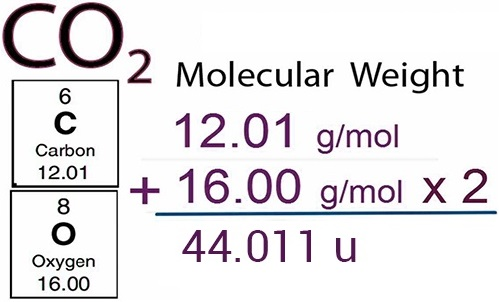Find free online Chemistry Topics covering a broad range of concepts from research institutes around the world.

## Molar Mass – Definition, Formula

Similar to relative atomic mass, relative molecular mass is defined as the ratio of the mass of a molecule to the unified atomic mass unit. The relative molecular mass of any compound can be calculated by adding the relative atomic masses of its constituent atoms.For example,

(i) Relative molecular mass of hydrogen molecule (H2)
= 2 × (relative atomic mass of hydrogen atom)
= 2 × 1.008 u
= 2.016 u.

(ii) Relative molecular mass of glucose (C6H12O6)
= (6 × 12) + ( 12 × 1.008) + (6 × 16)
= 72 + 12.096 + 96
= 180.096 u

Relative Atomic Masses of Some Elements

 Element Relative atomic mass Element Relative atomic mass H 1.008 Cl 35.45 C 12 K 39.10 N 14 Ca 40.08 O 16 Cr 51.99 Na 23 Mn 54.94 Mg 24.3 Fe 55.85 S 32.07 Cu 63.55